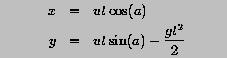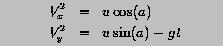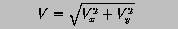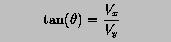# Programming Example: Projectile Motion### Problem Statement

This program computes the position (x and y coordinates) and the velocity (magnitude and direction) of a projectile, given t, the time since launch, u, the launch velocity, a, the initial angle of launch (in degree), and g=9.8, the acceleration due to gravity.

The horizontal and vertical displacements are given by the following formulae:The horizontal and vertical components of the velocity vector are computed asand the magnitude of the velocity vector isFinally, the angle between the ground and the velocity vector is determined by the formula below:Write a program to read in the launch angle a, the time since launch t, and the launch velocity u, and compute the position, the velocity and the angle with the ground.### Solution

```! --------------------------------------------------------------------
! Given t, the time since launch, u, the launch velocity, a, the
! initial angle of launch (in degree), and g, the acceleration due to
! gravity, this program computes the position (x and y coordinates)
! and the velocity (magnitude and direction) of a projectile.
! --------------------------------------------------------------------

PROGRAM  Projectile
IMPLICIT    NONE

REAL, PARAMETER :: g  = 9.8          ! acceleration due to gravity
REAL, PARAMETER :: PI = 3.1415926    ! you knew this.  didn't you

REAL            :: Angle             ! launch angle in degree
REAL            :: Time              ! time to flight
REAL            :: Theta             ! direction at time in degree
REAL            :: U                 ! launch velocity
REAL            :: V                 ! resultant velocity
REAL            :: Vx                ! horizontal velocity
REAL            :: Vy                ! vertical velocity
REAL            :: X                 ! horizontal displacement
REAL            :: Y                 ! vertical displacement

Angle = Angle * PI / 180.0           ! convert to radian
X     = U * COS(Angle) * Time
Y     = U * SIN(Angle) * Time - g*Time*Time / 2.0
Vx    = U * COS(Angle)
Vy    = U * SIN(Angle) - g * Time
V     = SQRT(Vx*Vx + Vy*Vy)
Theta = ATAN(Vy/Vx) * 180.0 / PI

WRITE(*,*)  'Horizontal displacement : ', X
WRITE(*,*)  'Vertical displacement   : ', Y
WRITE(*,*)  'Resultant velocity      : ', V
WRITE(*,*)  'Direction (in degree)   : ', Theta

END PROGRAM  Projectile
```
Click here to download this program.### Program Output

If the input to the program consists of the following three real values:
```45.0  6.0  60.0
```

The program will generate the following output:

```Horizontal displacement : 254.558472
Vertical displacement   : 78.158432
Resultant velocity      : 45.4763107
Direction (in degree)   : -21.1030636
```### Discussion

• The program uses Angle for the angle a, Time for t, and U for u. The READ statement reads the input.
• The first assignment statement converts the angle in degree to radian. This is necessary since all intrinsic trigonometric functions use radian rather than degree.
• Variables X and Y, which are computed in the second and third assignments, hold the displacements.
• The next two assignments compute the components of the velocity vector.
• The velocity itself is computed in the sixth assignment.
• Finally, the angle with ground, Theta, is computed with the last assignment. Note that ithe result is converted back to degree, since ATAN(x) returns the arc tangent value of x in radian.